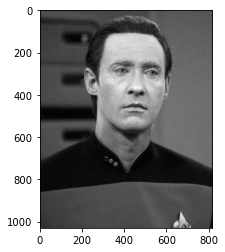Low rank approximation singular value decomposition for image compression

``````import numpy as np
import matplotlib.pyplot as plt
import matplotlib.image as mpimg
import scipy.linalg as la
``````
``````def LowRankApprox(A,k): #Uses linalg svd to return an approximation to A of rank k

# First, retrieve the SVD decomposition of A

# Take the vector of singular values, copy it, and kill all but the first k entries of the copy

Sigma_cutoff = Sigma
for i in range(len(Sigma)-k):
Sigma_cutoff[k+i] = 0 #(Sigma_k in the notes)

# Compute a new matrix using the cut-off singular values, and return it as value
return np.dot(U*Sigma,Vadj) #This A_k = U times Sigma_k times V^* in our notes
``````
``````def sz(k):
return 1030*k+k+816*k
``````

Image to Approximate

``````M = mpimg.imread('data.jpg') #Reads an RGB image, which is made out of 3 MxN matrices
picplot = plt.imshow(M)
M.shape
``````
``````(1030, 816, 3)
``````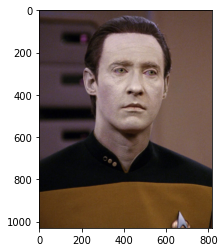Gray scale, so we don’t have to think about the RGB values

``````Mgs = np.mean(M, axis=2) #Create a grayscale image by averaging the colors of the 3 matrices
Mgs.shape
1030*816 #Original size of the grayscaled file
``````
``````840480
``````
``````picplot = plt.imshow(Mgs, cmap = 'gray')
``````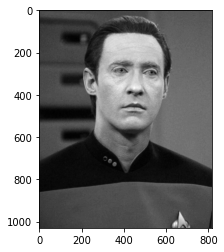VERY low rank approximation

``````rank = 1 # Set the rank of your approximation
B = LowRankApprox(Mgs,rank) # Best rank k approximation to the grayscale image
picshow = plt.imshow(B,cmap ='gray') #Plot the image
sz(1)
``````
``````1847
``````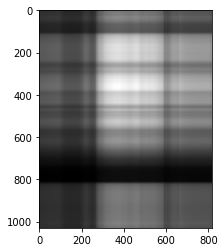Can’t see much of what’s going on. Can maybe make out a face, image size is very small

``````rank = 3
B = LowRankApprox(Mgs,rank)
picshow = plt.imshow(B,cmap ='gray')
sz(3)
``````
``````5541
``````Better, can definetly see a face

``````rank = 15
B = LowRankApprox(Mgs,rank)
picshow = plt.imshow(B,cmap ='gray')
sz(15)
``````
``````27705
``````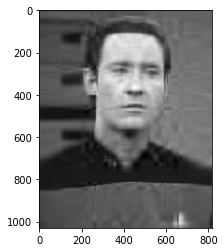Looks like data, even if blurry. Size is still heavily compressed

``````rank = 25
B = LowRankApprox(Mgs,rank)
picshow = plt.imshow(B,cmap ='gray')
sz(25)
``````
``````46175
``````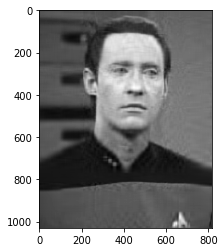``````rank = 80
B = LowRankApprox(Mgs,rank)
picshow = plt.imshow(B,cmap ='gray')
sz(80)
``````
``````147760
``````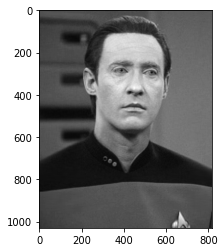Can’t determine the difference between these two images. Maybe slight bluring at the line formed on his uniform. We are about 1/8th the size of the original image.

``````picshow = plt.imshow(Mgs,cmap ='gray') # Original image
``````